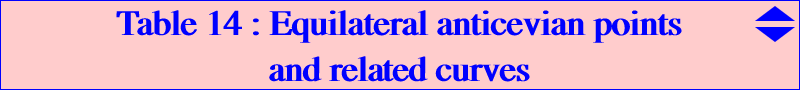This page was written using several insightful ideas by Jean-Pierre Ehrmann   A point P is an equilateral anticevian point if the anticevian (or precevian) triangle of P is equilateral. The corresponding center of the triangle is denoted by Q. Compare this page to table 10 and table 40 when "anticevian" is replaced with "cevian" and "circumanticevian". Let P' be the isotomic conjugate of the anticomplement of P = u : v : w. We have P' = 1 / (v + w - u) : : which we call the TA-transform of P. Conversely, P is called the CT-transform of P' since it is the complement of the isotomic conjugate of P'. The anticevian triangle of P is homothetic to the cevian triangle of P' and the center of homothety is the barycentric product of P and P'. Hence, a point P is an equilateral anticevian point if and only if P' is one of the six points X(370) and mates mentioned in table 10. It follows that there are six equilateral anticevian points P which lie on the CT-transform of any curve containing the six equilateral cevian points. These six points come in two groups of three points : the blue group always contains real points and the red group contains one real point and two other not necessarily real. I cannot resist to the pleasure of quoting Jean-Pierre Ehrmann (private email to Paul and myself) to explain the construction of these points.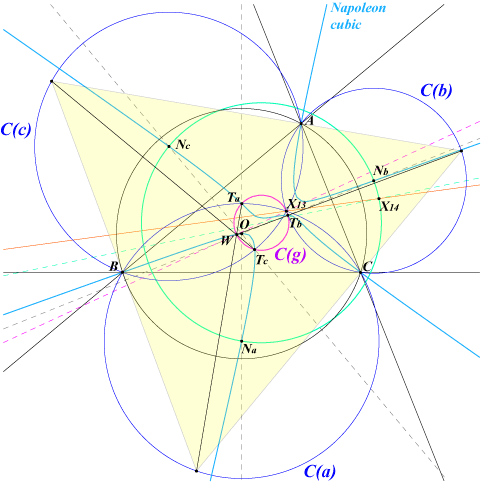Let F be one of the Fermat points X(13) (on the figure) or X(14) and NaNbNc the corresponding Napoleon triangle with vertices the circumcenters of the circles C(a) = FBC, C(b) = FCA, C(c) = FAB. The lines ONa, ONb, ONc intersect again the Napoleon cubic at Ta, Tb, Tc. These points lie on C(a), C(b), C(c) respectively and are the vertices of the other Napoleon triangle inscribed in the circle C(g) with center G going through F. C(g) is the locus of the centers of the equilateral circumscribed triangles directly similar with the pedal triangle of F*, the isogonal conjugate of F. For any point W on C(g), the vertices of the circumscribed equilateral triangle with center W are the second intersections of the lines WTa, WTb, WTc with C(a), C(b), C(c) respectively.Now, the centers of the precevian equilateral triangles directly similar with the pedal triangle of F* are the other common points - apart Ta, Tb, Tc - of the circle C(g) and the Napoleon cubic (only one such point appears on the figure). The corresponding perspectors lie on the isogonal pK with pivot the midpoint of F'F'* (F' is the other Fermat point) i.e. K129a or K129b (see below). A large number of curve are related to these six equilateral anticevian points P and the corresponding centers Q of the equilateral anticevian triangles.Conics and circles passing through the equilateral anticevian points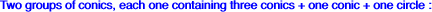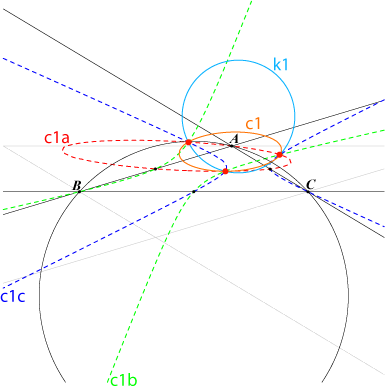c1a, c1b, c1c are the CT-transforms of the conics gamma_1a, gamma_1b, gamma_1c as seen in table 10. c1a contains A, the midpoints of AB and AC, the CT-transform A1 of A' reflection of A in BC (A1 lies on the line through K and the midpoint of BC and on the line through A and the foot of the orthic axis on BC). The tangent at A to c1a meets BC on the trilinear polar of X(13). c1a, c1b, c1c contain the red points and generate a net of conics which contains their "sum" c1 and a circle k1. c1 passes through X(13) and belongs to the pencil of conics generated by the inscribed Steiner ellipse and the polar circle. Its equation is : 2S (ST) + sqrt3 (PC) = 0 where : (ST) = x^2 - yz + cyclic (PC) = SA x^2 + cyclic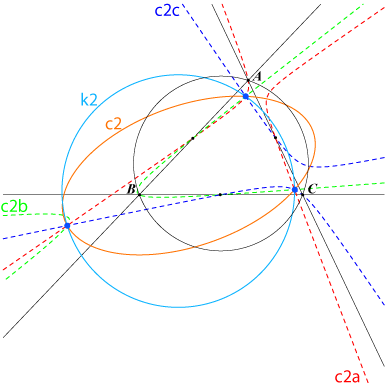Similarly, c2a, c2b, c2c are the CT-transforms of the conics gamma_2a, gamma_2b, gamma_2c as seen in table 10. c2a contains A, the midpoints of AB and AC, the CT-transform A1 of A'. The tangent at A to c2a meets BC on the trilinear polar of X(14). c2a, c2b, c2c contain the blue points and generate a net of conics which contains their "sum" c2 and a circle k2. c2 passes through X(14) and also belongs to the pencil of conics generated by the inscribed Steiner ellipse and the polar circle. Its equation is : 2S (ST) - sqrt3 (PC) = 0 (see above). Since the pencil of conics generated by c1 and c2 contains a circle (the polar circle), c1 and c2 have the same directions of axes.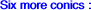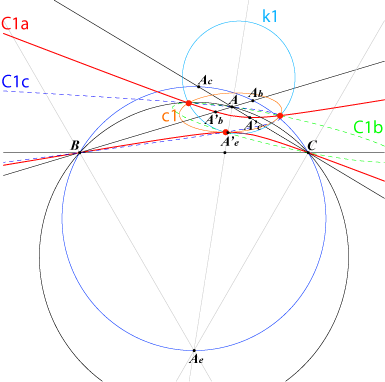C1a, C1b, C1c also belong to the net of conics generated by c1a, c1b, c1c but now each conic contains two vertices of ABC. C1a is in fact the locus of P such that its anticevian triangle PaPbPc has an 60°-angle at Pa. If Ae is the vertex of the equilateral triangle erected externally on BC, the circle BCAe meets AB, AC again at Ab, Ac. The harmonic conjugates A'b, A'c of Ab and Ac with respect to A, B and A, C respectively are two points on C1a. Similarly, the harmonic conjugate A'e of Ae with respect to A and the A-cevian of X(13) gives a fifth point and yields the construction of C1a. Note that the tangents at B, C to C1a, the line through K and the midpoint of BC, the A-cevian line of X(14) are concurrent.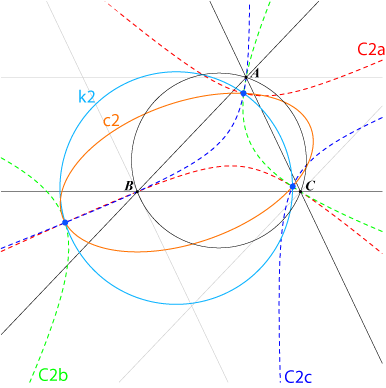C2a, C2b, C2c also belong to the net of conics generated by c2a, c2b, c2c and again each conic contains two vertices of ABC. The construction of C2a is similar to that of C1a but you will need an equilateral triangle erected internally on BC. Remark that C2a, C2b, C2c have always three real points in common when C1a, C1b, C1c may have only one real common point.Cubics passing through the equilateral anticevian pointsThe table below sums up different cubics through these red and blue points P :cubic CT-transform of ... points other points on the cubic K129a = pK(X6, X395) P X(1), X(2), X(6), X(14), X(16), X(18), X(62), X(395), X(1653) K129b = pK(X6, X396) P X(1), X(2), X(6), X(13), X(15), X(17), X(61), X(396), X(1652) pK(X395, X2) K264b = pK(X2, X298) P X(2), X(14), X(395), X(619) pK(X396, X2) K264a = pK(X2, X299) P X(2), X(13), X(396), X(618) K278 = pK(X1989, X1989) P, P X(2), X(13), X(14), X(395), X(396), X(1989) K277 K001 Neuberg cubic P, P X(6), X(37), X(216), X(395), X(396), X(1989)Remarks : There are two cubics which are both decomposed into three lines, each passing through either the red or blue points P. The equations are complicated. K129a and pK(X395, X2) generate a pencil of circum-cubics passing through the red points and X(2), X(14), X(395). K129b and pK(X396, X2) generate a pencil of circum-cubics passing through the blue points and X(2), X(13), X(396). K277 and K278 generate a pencil of cubics passing through the blue and red points and X(395), X(396), X(1989). K278 is a circum-cubic through the six points. In fact, K278 is a special case (it is a pK) of such cubics which can be defined as follows. Consider a point P and the Euler line Ep of its anticevian triangle. Paul Yiu noticed that Ep is parallel to the Euler line of ABC if and only if P lies on K278. Now, if Ep stays parallel to a fixed line, the locus of P is always a sextic which decomposes into the sidelines of the medial triangle and a circum-cubic through the six equilateral anticevian points. This cubic is a pK if and only if the line is the Euler line of ABC. It cannot be a circular cubic nor an equilateral cubic. It is obvious that all these cubics form a pencil of cubics.Higher degree curves passing through the equilateral anticevian points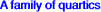Let P be a point and PaPbPc its anticevian triangle. PaPbPc is isosceles at Pa if and only if P lies on a quartic Qa. Similarly define Qb, Qc. These three quartics all contain A, B, C, the midpoints Ma, Mb, Mc of ABC and clearly the six equilateral anticevian points. Now, for a given center X = p : q : r, define the quartic Qx = p Qa + q Qb + r Qc which obviously passes through the same already mentioned twelve points. Qx meets BC again at a fourth point U, CA at V, AB at W. These points U, V, W are collinear if and only if X lies on the Neuberg cubic. In this case, the tangents at A, B, C concur at a point lying on the Napoleon cubic. Here is a short selection of such quartics :X points on the quartic remarks G X(2), X(216), X(395), X(396) O X(6), X(15), X(16), X(17), X(18), X(216) K X(1), X(2), X(3), excenters CT-transform of Q034 X(30) X(13), X(14), X(216), X(1989) see Q040